##### Microeconomics For Dummies - UK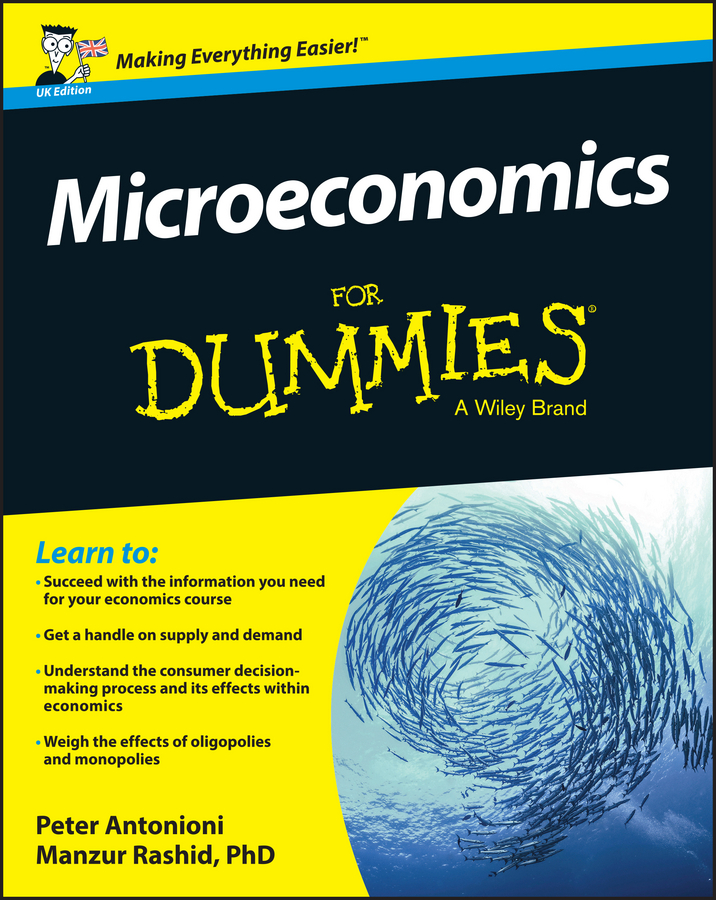The ultimate source of power in a market, even a monopolistic market, is the consumer, who still responds to price by changing his demand level. As a consumer, you get to decide whether you’re willing and able to purchase a good at a given price.

In theory, the monopolist can charge any price it wants, but practically, the monopolist can’t charge too high of a price or you won’t buy the good. The monopolist is constrained by your willingness to pay the price it charges.

For example, economists consider De Beers a resource monopoly because it effectively controls the world’s supply of diamonds. And although diamonds are very popular, if De Beers keeps raising its price, consumers will start substituting other precious gems, such as rubies and emeralds, for diamonds. Thus, as diamond prices increase, the quantity of diamonds consumers purchase will decrease.

The monopolist’s pricing decision is subject to the constraint imposed by consumer demand. If the monopolist charges too high of a price, nobody wants to buy its product. So, if the monopolist wants to sell more product, it must lower price as indicated by the market demand curve.

The inverse relationship between price and quantity demanded is the critical element in monopoly price setting. Because a single firm provides the entire quantity of the commodity in the market, the demand for the monopolist’s product, represented by a lower-case d, is the same as the market demand, represented by a capital D.

The market demand possesses the usual characteristics; an inverse relationship between price and quantity demanded and changing price elasticity of demand along the demand curve. In order to sell more of its product, the monopolist must lower its price, not only for the additional unit but for every other unit as well.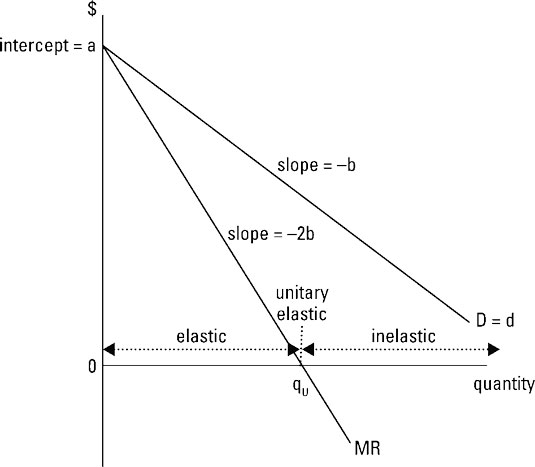The illustration also shows the relationship between a monopolist’s demand and marginal revenue. Remember that marginal revenue is the change in total revenue that occurs when one additional unit of a good is produced and sold. Because the monopolist’s demand curve is identical to the market demand curve, the monopolist can sell an additional unit of output only by lowering the product’s price.

Assuming no price discrimination (charging different customers different prices for the same good), this lower price is charged for all units of the commodity sold. As a consequence, the firm’s marginal revenue curve lies below its demand curve. Marginal revenue is less than price.

Marginal revenue — the change in total revenue — is below the demand curve.

Marginal revenue is related to the price elasticity of demand — the responsiveness of quantity demanded to a change in price. When marginal revenue is positive, demand is elastic; and when marginal revenue is negative, demand is inelastic. The output level at which marginal revenue equals zero corresponds to unitary elasticity. This occurs at the quantity qu in the illustration.

A linear demand curve has the form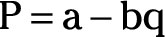where P is the good’s price in dollars and q is the quantity demanded. Constants in the equation are represented by a and ba is the intercept of the demand curve (where the demand curve intersects the vertical axis) and b is the demand curve’s slope.

Total revenue, TR, equals price times quantity or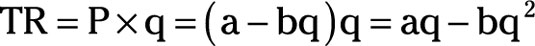Marginal revenue, MR, equals the derivative of total revenue taken with respect to quantity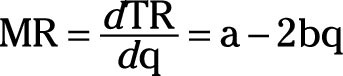If you compare the marginal revenue equation with the demand equation, you see that both equations have an intercept represented by a. The slope of the demand equation is represented by –b, while the slope of the marginal revenue equation is –2b. Thus, for a linear demand curve, the marginal revenue curve starts at the same intercept as the demand curve, but its slope is twice as steep.